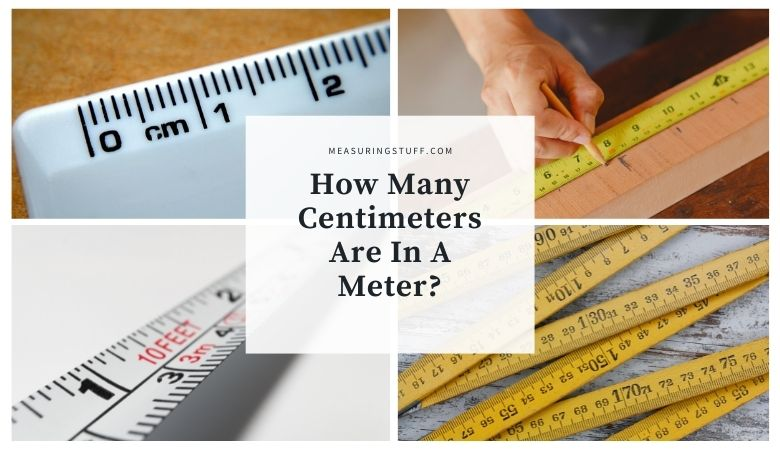# How Many Centimeters Are In A Meter?

Figuring out how many centimeters are in a meter may seem tricky, but there’s a simple formula that can quickly bring you to the answer.

There are 100 centimeters in a single meter.

To find out how many centimeters there are in any given number of meters, you can multiply the number of meters by 100.

The answer is how many centimeters are contained in the number of meters.

For example, if you want to find out how many centimeters are in 12 meters, you would multiply 12 by 100.

The answer is 1200, which tells you that there are 1200 centimeters in 12 meters.

If you want to convert centimeters into meters, you can use the opposite formula.

Instead of multiplying the number of meters by 100, divide the number of centimeters by 100.

For example, if you want to know how many meters there are in 1500 centimeters, divide 1500 by 100.

The answer, 15, tells you that 1500 centimeters equals 15 meters.

To calculate how many whole meters there are in a number of centimeters, you can also just move the decimal point back two spaces.

For example, if you have 1000 centimeters, move the decimal point two space to the left to get 10, which is the number of whole meters contained in 1000 centimeters.

You can also use the following table to convert meters into centimeters.

## Centimeters and Meters Measurement

Centimeters and meters are both units of measurement in the metric system, which is a general scale of measurement used around the world.

Because the system utilizes decimals and whole numbers as units of measurement increase, calculating the differences between centimeters, meters, and other measurements of length, such as millimeters or kilometers, is relatively straightforward.

## Meters To Centimeters Conversion Calculator

Use the following calculator to easily convert meters into centimeters.

For our complete section of unit conversions including tables and calculators click the link here.